Discrete Mathematics and Combinatorics Commons™

970 Full-Text Articles 1,129 Authors 104,022 Downloads94 Institutions

All Articles in Discrete Mathematics and Combinatorics

970 full-text articles. Page 8 of 36.

On The Inverse Of A Class Of Weighted Graphs, 2018Indian Statistical Institute - Delhi Center

On The Inverse Of A Class Of Weighted Graphs, Swarup Kumar Panda, Sukanta Pati

Electronic Journal of Linear Algebra

In this article, only connected bipartite graphs $G$ with a unique perfect matching $\c{M}$ are considered. Let $G_\w$ denote the weighted graph obtained from $G$ by giving weights to its edges using the positive weight function $\w:E(G)\ar (0,\ity)$ such that $\w(e)=1$ for each $e\in\c{M}$. An unweighted graph $G$ may be viewed as a weighted graph with the weight function $\w\equiv\1$ (all ones vector). A weighted graph $G_\w$ is nonsingular if its adjacency matrix $A(G_\w)$ is nonsingular. The {\em inverse} of a nonsingular weighted graph ...

On The Second Least Distance Eigenvalue Of A Graph, 2018College of Mathematics and Systems Science, Xinjiang University, Urumqi, Xinjiang 830046, P.R.China.

On The Second Least Distance Eigenvalue Of A Graph, Xueyi Huang, Qiongxiang Huang, Lu Lu

Electronic Journal of Linear Algebra

Let $G$ be a connected graph on $n$ vertices, and let $D(G)$ be the distance matrix of $G$. Let $\partial_1(G)\ge\partial_2(G)\ge\cdots\ge\partial_n(G)$ denote the eigenvalues of $D(G)$. In this paper, the connected graphs with @n􀀀1(G) at least the smallest root of $x^3=3x^2-11x-6 = 0$ are determined. Additionally, some non-isomorphic distance cospectral graphs are given.

Traveling In Networks With Blinking Nodes, 2018Southern CT State University

Traveling In Networks With Blinking Nodes, Braxton Carrigan, James Hammer

Theory and Applications of Graphs

We say that a blinking node system modulo $n$ is an ordered pair $(G,L)$ where $G$ is a graph and $L$ is an on-labelling which indicates when vertices can be visited. An On-Hamiltonian walk is a sequence of all the vertices of $G$ such that the position of each vertex modulo $n$ is an integer of the label of that vertex. This paper will primarily investigate finding the shortest On-Hamiltonian walks in a blinking node system on complete graphs and complete bipartite graphs but also establishes the terminology and initial observations for working with blinking node systems on other ...

The Graphs And Matroids Whose Only Odd Circuits Are Small, 2018Louisiana State University and Agricultural and Mechanical College

The Graphs And Matroids Whose Only Odd Circuits Are Small, Kristen Nicole Wetzler

LSU Doctoral Dissertations

This thesis is motivated by a graph-theoretical result of Maffray, which states that a 2-connected graph with no odd cycles exceeding length 3 is bipartite, is isomorphic to K_4, or is a collection of triangles glued together along a common edge. We first prove that a connected simple binary matroid M has no odd circuits other than triangles if and only if M is affine, M is M(K_4) or F_7, or M is the cycle matroid of a graph consisting of a collection of triangles glued together along a common edge. This result implies that a 2-connected loopless graph ...

2018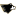Michigan Technological University

On The Density Of The Odd Values Of The Partition Function, Samuel Judge

Dissertations, Master's Theses and Master's Reports

The purpose of this dissertation is to introduce a new approach to the study of one of the most basic and seemingly intractable problems in partition theory, namely the conjecture that the partition function $p(n)$ is equidistributed modulo $2$. We provide a doubly-indexed, infinite family of conjectural identities in the ring of series $\Z_2[[q]]$, which relate $p(n)$ with suitable $t$-multipartition functions, and show how to, in principle, prove each such identity. We will exhibit explicit proofs for $32$ of our identities. However, the conjecture remains open in full generality. A striking consequence of these conjectural identities ...

2018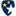Murray State University

Distributive Lattice Models Of The Type C One-Rowed Weyl Group Symmetric Functions, William Atkins

Murray State Theses and Dissertations

We present two families of diamond-colored distributive lattices – one known and one new – that we can show are models of the type C one-rowed Weyl symmetric functions. These lattices are constructed using certain sequences of positive integers that are visualized as ﬁlling the boxes of one-rowed partition diagrams. We show how natural orderings of these one-rowed tableaux produce our distributive lattices as sublattices of a more general object, and how a natural coloring of the edges of the associated order diagrams yields a certain diamond-coloring property. We show that each edge-colored lattice possesses a certain structure that is associated with ...

On The Domination Chain Of M By N Chess Graphs, 2018Murray State University

On The Domination Chain Of M By N Chess Graphs, Kathleen Johnson

Murray State Theses and Dissertations

A survey of the six domination chain parameters for both square and rectangular chess boards are discussed.

A Combinatorial Miscellany: Antipodes, Parking Cars, And Descent Set Powers, 2018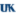University of Kentucky

A Combinatorial Miscellany: Antipodes, Parking Cars, And Descent Set Powers, Alexander Thomas Happ

Theses and Dissertations--Mathematics

In this dissertation we first introduce an extension of the notion of parking functions to cars of different sizes. We prove a product formula for the number of such sequences and provide a refinement using a multi-parameter extension of the Abel--Rothe polynomial. Next, we study the incidence Hopf algebra on the noncrossing partition lattice. We demonstrate a bijection between the terms in the canceled chain decomposition of its antipode and noncrossing hypertrees. Thirdly, we analyze the sum of the 𝑟th powers of the descent set statistic on permutations and how many small prime factors occur in these numbers. These results ...

2018Georgia Southern University

A General Lower Bound On Gallai-Ramsey Numbers For Non-Bipartite Graphs, Colton Magnant

Theory and Applications of Graphs

Given a graph $H$ and a positive integer $k$, the $k$-color Gallai-Ramsey number $gr_{k}(K_{3} : H)$ is defined to be the minimum number of vertices $n$ for which any $k$-coloring of the complete graph $K_{n}$ contains either a rainbow triangle or a monochromatic copy of $H$. The behavior of these numbers is rather well understood when $H$ is bipartite but when $H$ is not bipartite, this behavior is a bit more complicated. In this short note, we improve upon existing lower bounds for non-bipartite graphs $H$ to a value that we conjecture to be sharp ...

Pbw Bases And Marginally Large Tableaux In Type D, 2018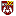Central Michigan University

Pbw Bases And Marginally Large Tableaux In Type D, Ben Salisbury, Adam Schultze, Peter Tingley

Mathematics and Statistics: Faculty Publications and Other Works

We give an explicit description of the unique crystal isomorphism between two realizations of B(∞) in type D: that using marginally large tableaux and that using PBW monomials with respect to one particularly nice reduced expression of the longest word

A Survey On Monochromatic Connections Of Graphs, 2018Nankai University

A Survey On Monochromatic Connections Of Graphs, Xueliang Li, Di Wu

Theory and Applications of Graphs

The concept of monochromatic connection of graphs was introduced by Caro and Yuster in 2011. Recently, a lot of results have been published about it.
In this survey, we attempt to bring together all the results that dealt with it.
We begin with an introduction, and then classify the results into the following categories: monochromatic connection coloring of edge-version, monochromatic connection coloring of vertex-version, monochromatic index, monochromatic connection coloring of total-version.

2018University of Kentucky

Hilbert Bases, Descent Statistics, And Combinatorial Semigroup Algebras, Mccabe J. Olsen

Theses and Dissertations--Mathematics

The broad topic of this dissertation is the study of algebraic structure arising from polyhedral geometric objects. There are three distinct topics covered over three main chapters. However, each of these topics are further linked by a connection to the Eulerian polynomials.

Chapter 2 studies Euler-Mahonian identities arising from both the symmetric group and generalized permutation groups. Specifically, we study the algebraic structure of unit cube semigroup algebra using Gröbner basis methods to acquire these identities. Moreover, this serves as a bridge between previous methods involving polyhedral geometry and triangulations with descent bases methods arising in representation theory.

In Chapter ...

Polytopes Associated To Graph Laplacians, 2018University of Kentucky

Polytopes Associated To Graph Laplacians, Marie Meyer

Theses and Dissertations--Mathematics

Graphs provide interesting ways to generate families of lattice polytopes. In particular, one can use matrices encoding the information of a finite graph to define vertices of a polytope. This dissertation initiates the study of the Laplacian simplex, PG, obtained from a finite graph G by taking the convex hull of the columns of the Laplacian matrix for G. The Laplacian simplex is extended through the use of a parallel construction with a finite digraph D to obtain the Laplacian polytope, PD.

Basic properties of both families of simplices, PG and PD, are established using techniques ...

Fine Structure Of 4-Critical Triangle-Free Graphs Iii. General Surfaces, 2018Charles University, Prague

Fine Structure Of 4-Critical Triangle-Free Graphs Iii. General Surfaces, Zdenek Dvorak, Bernard Lidicky

Mathematics Publications

Dvořák, Král', and Thomas [Three-Coloring Triangle-Free Graphs on Surfaces IV. Bounding Face Sizes of 4-Critical Graphs, preprint, arXiv:1404.6356v3, 2015; Three-Coloring Triangle-Free Graphs on Surfaces VI. 3-Colorability of Quadrangulations, preprint, arXiv:1509.01013, 2015] gave a description of the structure of triangle-free graphs on surfaces with respect to 3-coloring. Their description, however, contains two substructures (both related to graphs embedded in a plane with two precolored cycles) whose coloring properties are not entirely determined. In this paper, we fill these gaps.

3-Maps And Their Generalizations, 2018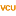Virginia Commonwealth University

3-Maps And Their Generalizations, Kevin J. Mccall

Theses and Dissertations

A 3-map is a 3-region colorable map. They have been studied by Craft and White in their paper 3-maps. This thesis introduces topological graph theory and then investigates 3-maps in detail, including examples, special types of 3-maps, the use of 3-maps to find the genus of special graphs, and a generalization known as n-maps.

2018University of Colorado, Boulder

Uses Of Mathematics In Computer Animation And 3d Rendering Software, Peter Rock

As the title suggests, this paper discusses the applications of several mathematical concepts to computer animation software generally used in the creation of movies and video games. Topics covered will include differential forms, conformal maps, surface texturing, and lighting techniques. It is not the goal of this paper to present anything particularly novel to the mathematical community, but rather to present something that is entertaining to read that will hopefully engage both mathematicians and sane people alike. This paper has been carefully crafted so that it should be accessible to most people with a Calc. I background. That being said ...

Extensions Of The Morse-Hedlund Theorem, 2018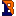Bucknell University

Extensions Of The Morse-Hedlund Theorem, Eben Blaisdell

Honors Theses

Bi-infinite words are sequences of characters that are infinite forwards and backwards; for example "...ababababab...". The Morse-Hedlund theorem says that a bi-infinite word f repeats itself, in at most n letters, if and only if the number of distinct subwords of length n is at most n. Using the example, "...ababababab...", there are 2 subwords of length 3, namely "aba" and "bab". Since 2 is less than 3, we must have that "...ababababab..." repeats itself after at most 3 letters. In fact it does repeat itself every two letters. Interestingly, there are many extensions of this theorem to multiple dimensions ...

Spn Graphs And Rank-1 Cp-Completable Graphs, 2018Iowa State University

Spn Graphs And Rank-1 Cp-Completable Graphs, Leslie Hogben, Naomi Shaked-Monderer

Mathematics Publications

A simple graph G is an SPN graph if every copositive matrix having graph G is the sum of a positive semidefinite and nonnegative matrix. SPN graphs were introduced in [Shaked-Monderer, SPN graphs: When copositive = SPN, Linear Algebra Appl., 509(15):82-113, 2016], where it was conjectured that the complete subdivision graph of K4 is an SPN graph. We disprove this conjecture, which in conjunction with results in the Shaked-Monderer paper show that a subdivision of K4 is a SPN graph if and only if at most one edge is subdivided. We conjecture that a graph is an SPN graph ...

Zero Forcing And Power Domination For Graph Products, 2018Westminster College - Fulton

Zero Forcing And Power Domination For Graph Products, Katherine F. Benson, Daniela Ferrero, Mary Flagg, Veronika Furst, Leslie Hogben, Violeta Vasilevska, Brian Wissman

Mathematics Publications

The power domination number arose from the monitoring of electrical networks, and methods for its determination have the associated application. The zero forcing number arose in the study of maximum nullity among symmetric matrices described by a graph (and also in control of quantum systems and in graph search algorithms). There has been considerable effort devoted to the determination of the power domination number, the zero forcing number, and maximum nullity for specific families of graphs. In this paper we exploit the natural relationship between power domination and zero forcing to obtain results for the power domination number of tensor ...

Polychromatic Colorings On The Hypercube, 2018West Virginia University

Polychromatic Colorings On The Hypercube, John Goldwasser, Bernard Lidicky, Ryan R. Martin, David Offner, John Talbot, Michael Young

Mathematics Publications

Given a subgraph G of the hypercube Qn, a coloring of the edges of Qn such that every embedding of G contains an edge of every color is called a G-polychromatic coloring. The maximum number of colors with which it is possible to G-polychromatically color the edges of any hypercube is called the polychromatic number of G. To determine polychromatic numbers, it is only necessary to consider a specific type of coloring, which we call simple. The main tool for finding upper bounds on polychromatic numbers is to translate the question of polychromatically coloring the hypercube so every embedding of ...